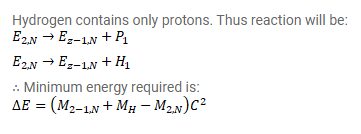# Show that the minimum energy needed to separate a proton from

Question:

Show that the minimum energy needed to separate a proton from a nucleus with Z protons and $\mathrm{N}$ neutrons is

$\Delta \mathrm{E}=\left(\mathrm{M}_{\mathrm{Z}-1, \mathrm{n}}+\mathrm{M}_{\mathrm{H}}-\mathrm{M}_{\mathrm{Z}, \mathrm{N}}\right) \mathrm{c}^{2}$

Where $M_{Z, N}=$ mass of an atom with $Z$ protons and $N$ neutrons in the nucleus and $M_{H}=$ mass of a hydrogen atom. This energy is known as proton-separation energy.

Solution: